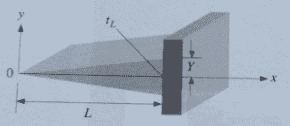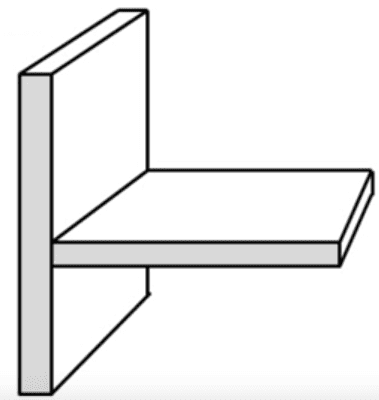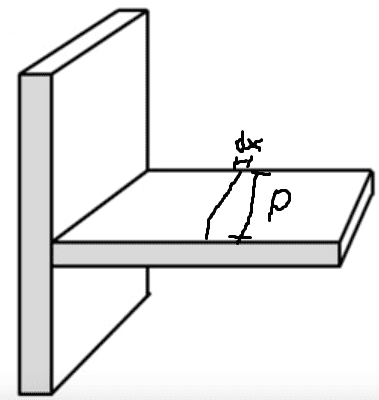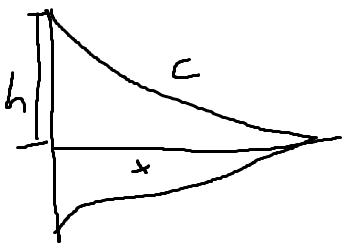Fin/Extended surface differential equation for temperature

I'm trying to deduce the differential equation for temperature for a triangular fin:I know that for a rectangular fin, such as:I can do:
Energy entering the left:
$$q_x= -kA\frac{dT(x)}{dx}$$

Energy leaving the right:
$$q_{x+dx} = -kA\frac{dT(x)}{dx} - kA\frac{d² T(x)}{dx²}dx$$

Energy lost by convection:
$$dq_{conv} = h_eA(T-T_e)$$
$$dq_{conv} = h_eP(T-T_e)dx$$

$$q_x - q_{x+dx} - dq_{conv} = 0$$

$$q_x = q_{x+dx} + dq_{conv}$$

$$-kA\frac{dT(x)}{dx} = -kA\frac{dT(x)}{dx} - kA\frac{d²T(x)}{dx²}dx + h_eP(T-T_e)dx$$

$$kA\frac{d²T(x)}{dx²}dx = h_eP(T-T_e)dx$$

$$\frac{d²T(x)}{dx²} = \frac{h_eP(T-T_e)}{kA}$$

But I don't understand why A = Pdx on the energy lost by convection, so I don't know how to adapt that for a triangular fin.

Seems to me that T(x,y,z) still changes predominantly on the x axis, so I assume the other two equations remain unchanged.

Chestermiller
Mentor
There are two areas involved here, one perpendicular to the flow of heat in the x direction (which you call A), and the other perpendicular to the flow of heat from the fin surface into the surrounding air (which is Pdx). For a fin with triangular shape, A is decreasing with x, and P is about constant. Also, since A is a function of x, when you do your heat balance on a differential section of fin, the area A must stay inside the heat flux derivative.

Chet

•roughwinds
There are two areas involved here, one perpendicular to the flow of heat in the x direction (which you call A), and the other perpendicular to the flow of heat from the fin surface into the surrounding air (which is Pdx). For a fin with triangular shape, A is decreasing with x, and P is about constant. Also, since A is a function of x, when you do your heat balance on a differential section of fin, the area A must stay inside the heat flux derivative.

Chet
So this is correct?

$$q_x = -kA(x)\frac{dT(x) }{dx}$$
$$q_{x+dx} = -kA(x)\frac{dT(x) }{dx} - k\frac{dA(x)}{dx}\frac{d²T(x)}{dx²}$$
$$dq_{conv} = h_eP(T(x)-T_e)dx$$
$$-kA(x)\frac{dT(x) }{dx} = -kA(x)\frac{dT(x) }{dx} - k\frac{dA(x)}{dx}\frac{d²T(x)}{dx²} + h_eP(T(x)-T_e)dx$$
$$k\frac{dA(x)}{dx}\frac{d²T(x)}{dx²} = h_eP(T(x)-T_e)dx$$
$$\frac{dA(x)}{dx}\frac{d²T(x) }{dx²} = \frac{h_eP(T(x)-T_e)}{k}$$
$$T(x)-T_e = θ(x)$$
$$m² = \frac{h_eP}{k}$$
$$\frac{d²T(x)}{dx²} = \frac{d²θ(x)}{dx²}$$
$$\frac{dA(x)}{dx}\frac{d²θ(x) }{dx²} = m²θ(x)$$ (1)

What I've posted here is just a portion of an exercise I was doing, I would then have to solve the ODE to find an equation for T(x). So now I need to get rid of this derivative of A(x). To be honest the only thing I can think of to proceed from here is to find an equation for A(x) and derivate it.$$A(x) = 2h(x)P$$
$$c² = h² + x²$$
$$h(x) = sqrt(c²-x²)$$
$$A(x) = 2sqrt(c²-x²)P$$
$$\frac{dA(x)}{dx} = \frac{-2Px}{sqrt(c²-x²)}$$
$$\frac{d²θ(x) }{dx²} = -\frac{m²θ(x)sqrt(c²-x²)}{2Px}$$

And this is feeling too awkward to be correct, lol. Can you integrate back at (1)?
------
Just thought of
$$n²(x) = -\frac{m²sqrt(c²-x²)}{2Px}$$
$$\frac{d²θ(x)}{dx²} = n²(x)θ(x)$$
$$\frac{d²θ(x)}{dx²} - n²(x)θ(x) = 0$$
$$θ(x) = c_1e^{nx} + c_2e^{-nx}$$
I know how to continue from here, but I'm having the sensation that this is wrong.

Last edited:
Chestermiller
Mentor
If P is the dimension shown on your diagram, then the differential heat balance should read:
$$k\frac{\partial}{\partial x}\left(A(x)\frac{\partial T}{\partial x}\right)-2Ph(T-T_e)=0$$
Also, from the figure, A(x) = P t(x), where t is the thickness of the fin at location x. So:
$$k\frac{\partial}{\partial x}\left(t(x)\frac{\partial T}{\partial x}\right)-2h(T-T_e)=0$$

•roughwinds
If P is the dimension shown on your diagram, then the differential heat balance should read:
$$k\frac{\partial}{\partial x}\left(A(x)\frac{\partial T}{\partial x}\right)-2Ph(T-T_e)=0$$
Also, from the figure, A(x) = P t(x), where t is the thickness of the fin at location x. So:
$$k\frac{\partial}{\partial x}\left(t(x)\frac{\partial T}{\partial x}\right)-2h(T-T_e)=0$$
I don't think I expressed myself properly before but anyway, finally managed to get it in the way I wanted:
http://i.share.pho.to/0a247f78_o.jpeg
http://i.share.pho.to/57739467_o.jpeg
$$T(x) - T_e = θ(x)$$
$$m = \frac{h_e(L/e)(1+(e/L)²)^{1/2}}{k}$$
$$xθ'' + θ' - mθ = 0$$
Just need to solve that now.

Chestermiller
Mentor
I don't think I expressed myself properly before but anyway, finally managed to get it in the way I wanted:
http://i.share.pho.to/0a247f78_o.jpeg
http://i.share.pho.to/57739467_o.jpeg
$$T(x) - T_e = θ(x)$$
$$m = \frac{h_e(L/e)(1+(e/L)²)^{1/2}}{k}$$
$$xθ'' + θ' - mθ = 0$$
Just need to solve that now.
This is an Euler differential equation.

This is an Euler differential equation.
Wouldn't it have to be
$$x²θ′′+xθ′−mθ=0$$
for it to be an Euler differential equation?

I tried to do power series but I got stuck in the end:
$$y=\sum_{n=0}^\infty a_k x^k$$
$$θ'=\sum_{n=1}^\infty ka_k x^{k-1}$$
$$θ''=\sum_{n=2}^\infty k(k-1)a_k x^{k-2}$$
$$\sum_{n=2}^\infty k(k-1)a_k x^{k-1} + \sum_{n=1}^\infty ka_k x^{k-1} - \sum_{n=0}^\infty m²a_k x^k = 0$$
$$\sum_{n=1}^\infty k(k+1)a_{k+1} x^{k} + \sum_{n=0}^\infty (k+1)a_{k+1} x^{k} - \sum_{n=0}^\infty m²a_k x^k = 0$$
$$a_1 - m²a_o + \sum_{n=1}^\infty k(k+1)a_{k+1} x^{k} + \sum_{n=1}^\infty (k+1)a_{k+1} x^{k} - \sum_{n=1}^\infty m²a_k x^k = 0$$
$$a_1 - m²a_o = 0$$
$$a_1 = m²a_o$$
$$\sum_{n=1}^\infty k(k+1)a_{k+1} x^{k} + \sum_{n=1}^\infty (k+1)a_{k+1} x^{k} - \sum_{n=1}^\infty m²a_k x^k = 0$$
$$\sum_{n=1}^\infty[k(k+1)a_{k+1} x^{k} + (k+1)a_{k+1} x^{k} - m²a_k x^k]= 0$$
$$\sum_{n=1}^\infty[k(k+1)a_{k+1} + (k+1)a_{k+1} - m²a_k]x^{k}= 0$$
$$\sum_{n=1}^\infty[(k+1)(ka_{k+1} + a_{k+1}) - m²a_k]x^{k}= 0$$
$$\sum_{n=1}^\infty[(k+1)(k+1)a_{k+1} - m²a_k]x^{k}= 0$$
$$\sum_{n=1}^\infty[(k+1)²a_{k+1} - m²a_k]x^{k}= 0$$
$$(k+1)²a_{k+1} - m²a_k= 0$$
$$a_{k+1}= \frac{m²a_k}{(k+1)²}$$
$$k = 1, a_2 = \frac{m²a_1}{4} = \frac{m^{4}a_0}{2!}$$
$$k = 2, a_3 = \frac{m²a_2}{9} = \frac{m^{6}a_0}{(3!)²}$$
$$k = 3, a_4 = \frac{m²a_3}{16} = \frac{m^{8}a_0}{(4!)²}$$
$$k = 4, a_5 = \frac{m²a_4}{25} = \frac{m^{10}a_0}{(5!)²}$$
$$a_{2k}= \frac{m^{4k}a_0}{(2k!)²}$$
$$a_{2k+1}= \frac{m^{2(2k+1)}a_0}{((2k+1)!)²}$$
$$θ(x) = a_0\sum_{n=0}^\infty \frac{m^{4k}x^{2k}}{(2k!)²} + a_0\sum_{n=0}^\infty \frac{m^{2(2k+1)}x^{2k+1}}{((2k+1)!)²}$$

Chestermiller
Mentor
Wouldn't it have to be
$$x²θ′′+xθ′−mθ=0$$
for it to be an Euler differential equation?
Ooops. Sorry. My mistake.